## Goodness of fit in Rstudio

After estimating Ordinary Least Squares or OLS in Rstudio, we must ensure that the model is a good fit. The model must satisfy the assumptions of OLS including heteroscedasticity, no multicollinearity, normality of residuals and no autocorrelation. We can apply several tests and generate plots to check for the goodness of fit of a model.

Here, we will estimate the same OLS model as we did in the earlier post on OLS estimation. After this, we will illustrate the estimation of various tests and procedures essential for determining the fit of any OLS model. Our analysis will focus on the following:

• Checking the normality assumption of residuals using plots.
• Reporting the Standard errors, P-values, R-square, Adjusted R-square, Confidence intervals and Anova results.
• Testing for heteroscedasticity using several tests and plots.
• Check for the problem of multicollinearity.
• Perform tests of autocorrelation and generate Autocorrelation (ACF) and Partial autocorrelation (PACF) plots.

## Goodness of fit: code and results

We will use the “mtcars” data available in “datasets” package to estimate an OLS model. First step involves loading the libraries and the dataset, followed by the analysis.

Here we will illustrate the tests for Heterocedasticity, Multicollinearity and Autocorrelation that can be conducted easily using R or Rstudio.

OLS Estimation, Confidence Intervals, Anova and Normality of Residuals

The “lm” is used to estimate the OLS model. For Goodness of fit, the function automatically estimates Standard Errors, P-values, R-square and Adjusted R-square.

To supplement this, we can use confint to estimate the confidence intervals of the coefficients and anova to do an F-test.

Fitted values and residuals/error terms can be obtained using fitted and resid commands. To check the distribution of residuals graphically, we can plot a histogram using hist.

stargazer(ols1 <- lm(mpg ~ IV), type = "text", style = "all", title = "OLS results")
##
## OLS results
## ======================================================
##                            Dependent variable:
##                     ----------------------------------
##                                    mpg
## ------------------------------------------------------
## IVhp                              -0.018
##                                  (0.012)
##                                 t = -1.519
##                                 p = 0.141
## IVcyl                            -0.942*
##                                  (0.551)
##                                 t = -1.709
##                                 p = 0.099
## IVwt                            -3.167***
##                                  (0.741)
##                                 t = -4.276
##                                 p = 0.0002
## Constant                        38.752***
##                                  (1.787)
##                                 t = 21.687
##                                 p = 0.000
## ------------------------------------------------------
## Observations                        32
## R2                                0.843
## Residual Std. Error          2.512 (df = 28)
## F Statistic         50.171*** (df = 3; 28) (p = 0.000)
## ======================================================
## Note:                      *p<0.1; **p<0.05; ***p<0.01
conf <- confint(ols1, level = 0.95)
stargazer(conf, type = "text", title = "Confidence Intervals")
##
## Confidence Intervals
## =========================
##             2.5 %  97.5 %
## -------------------------
## (Intercept) 35.092 42.412
## IVhp        -0.042 0.006
## IVcyl       -2.070 0.187
## IVwt        -4.684 -1.650
## -------------------------
anova <- anova(ols1)
stargazer(anova, type = "text", title = "Anova")
##
## Anova
## ============================================
## Statistic N  Mean   St. Dev.   Min     Max
## --------------------------------------------
## Df        2 15.500   17.678     3      28
## Sum Sq    2 563.024 546.456  176.621 949.427
## Mean Sq   2 161.392 219.322   6.308  316.476
## F value   1 50.171           50.171  50.171
## Pr(> F)   1  0.000              0       0
## --------------------------------------------
ycap <- fitted(ols1)
error <- resid(ols1)
hist(error, breaks = 5)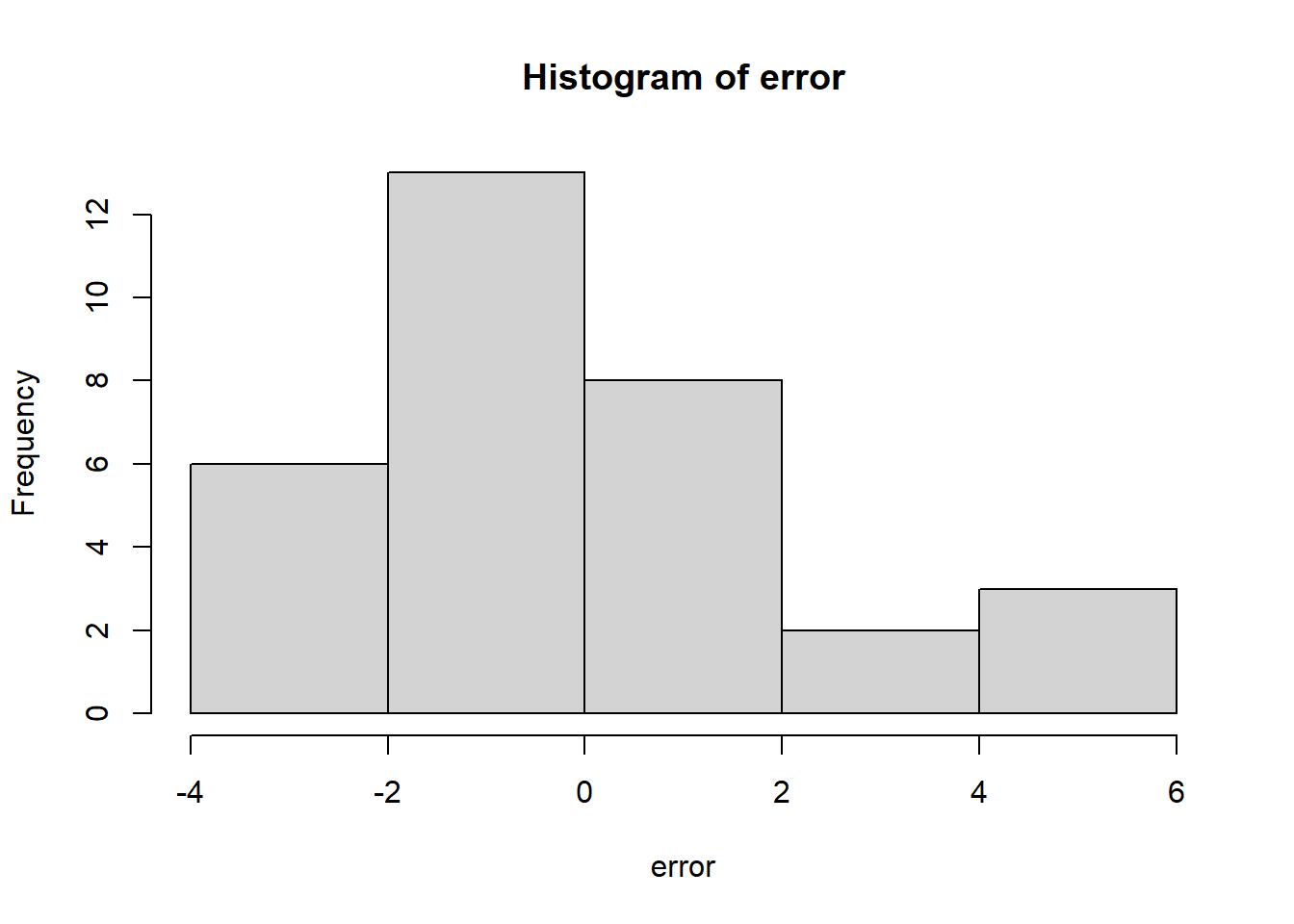Heteroscedasticity

We will use the skedastic package to apply various tests of heteroscedasticity.

We can also use the plot command to get some insights into the behaviour of residuals by plotting them with fitted values.

An interesting application of the plot command is to use it on the model object ols1 that we estimated: plot(ols1). This automatically generated 4 different types of plots that give insights into normality as well as heteroscedasticity.

plot(ycap,error) # Residual vs fitted plot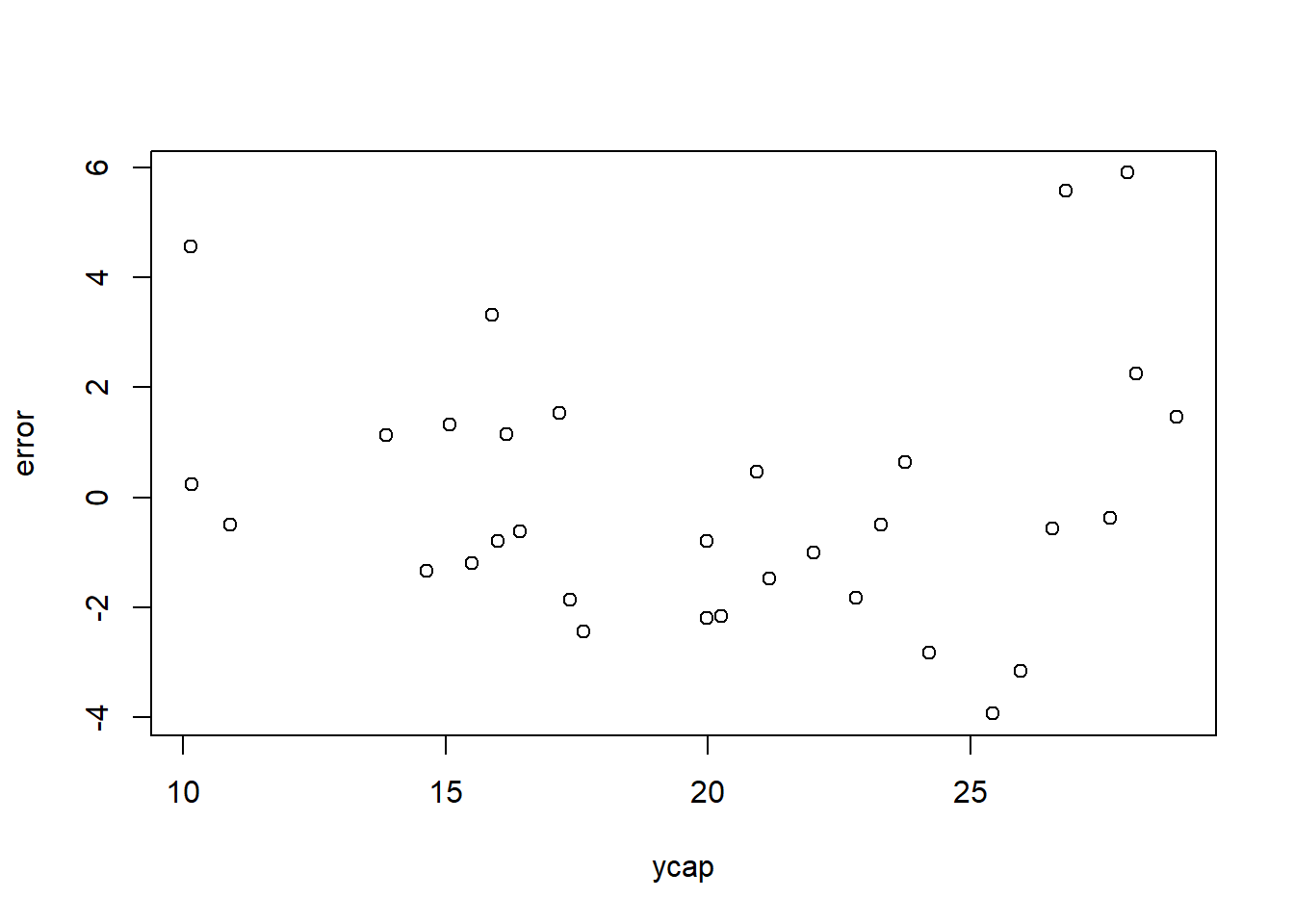plot(ols1) # 4 different types of plot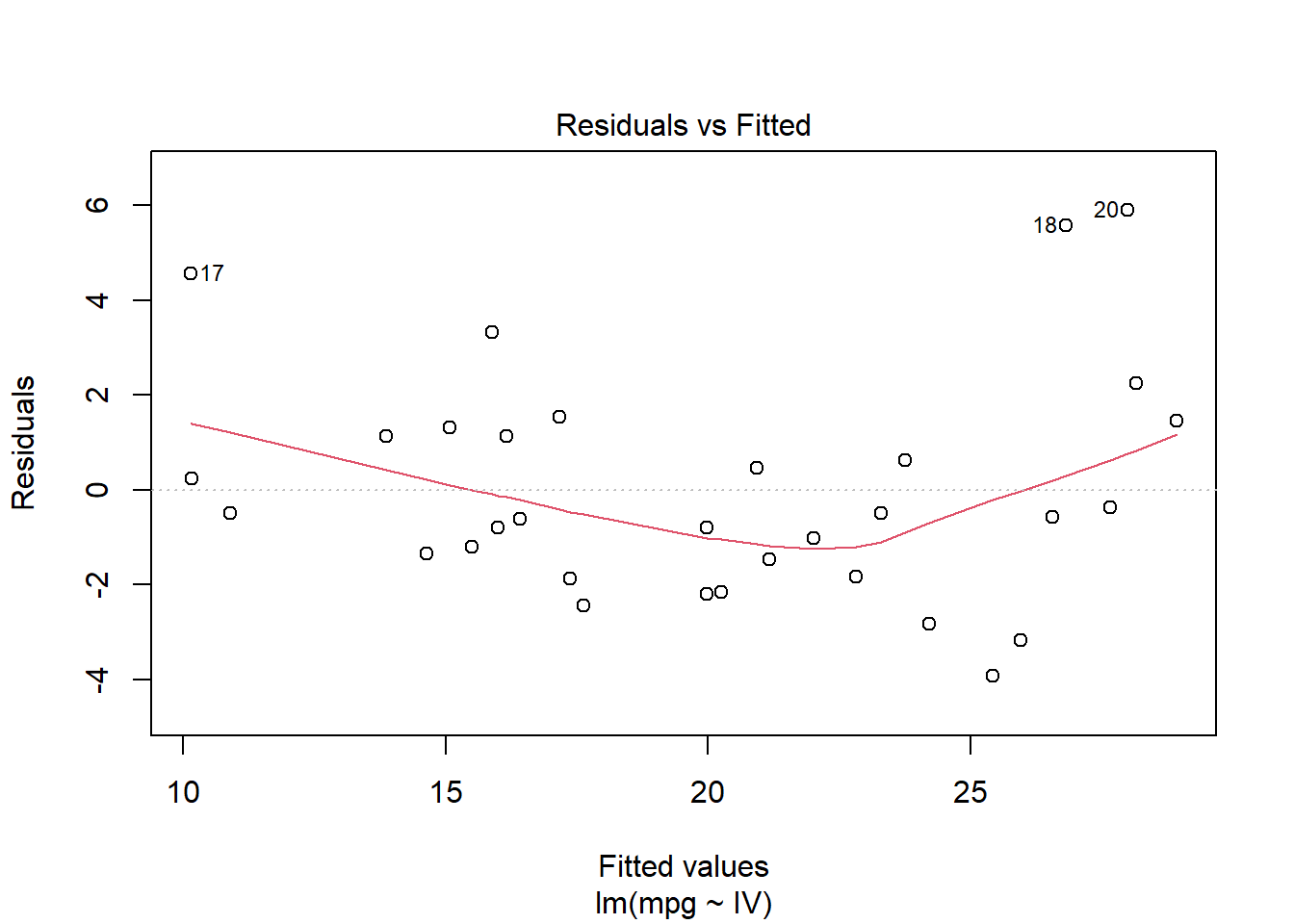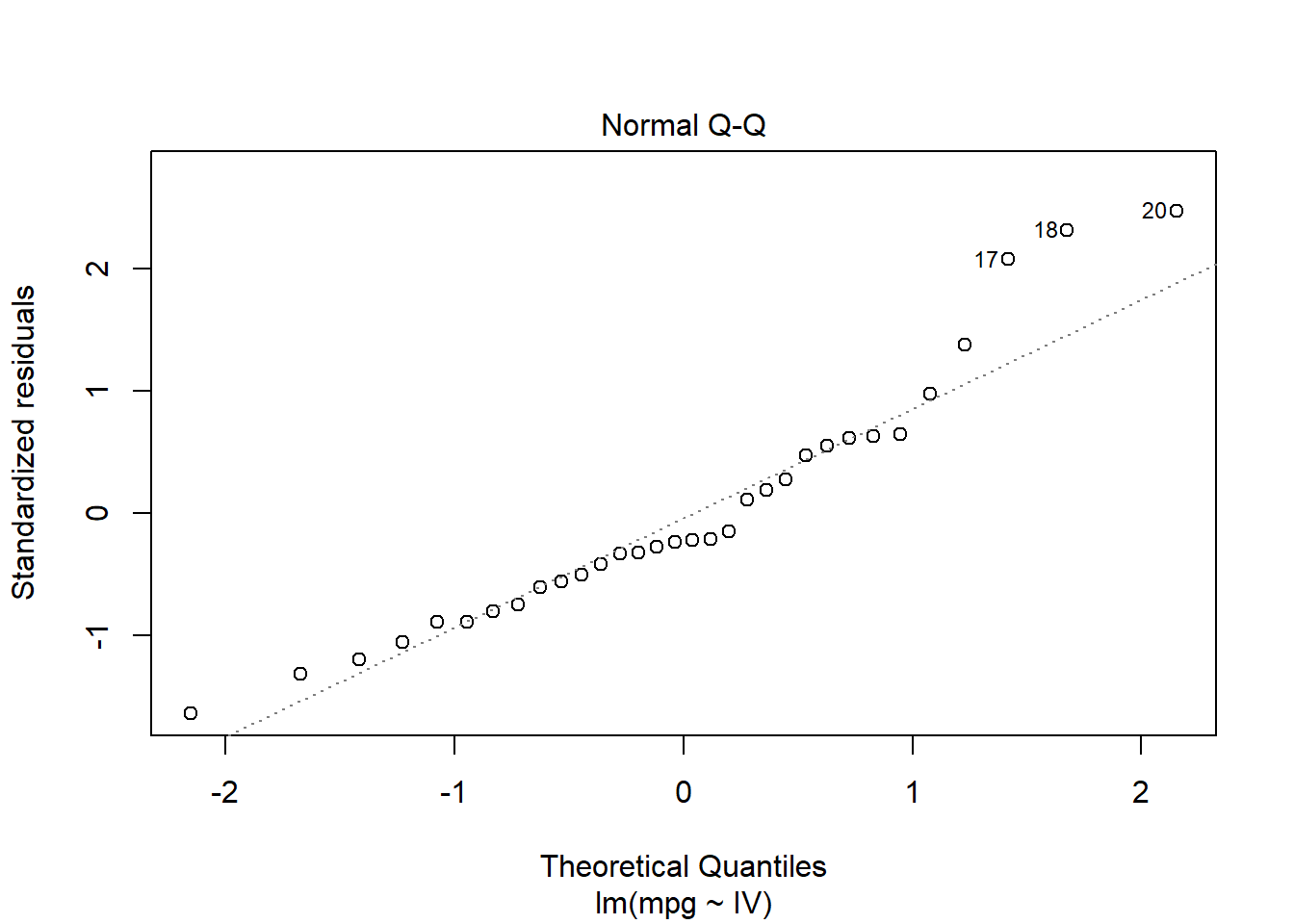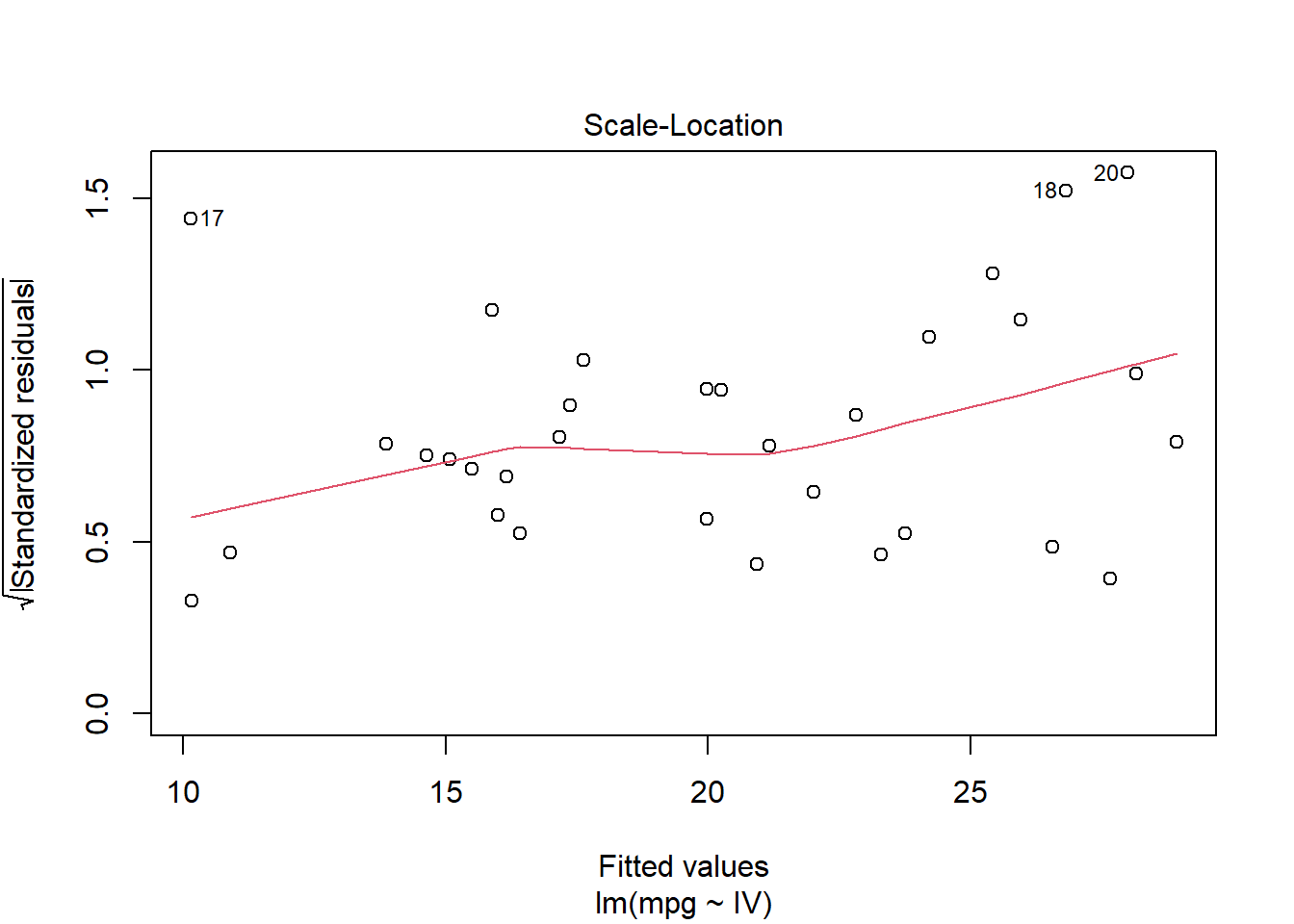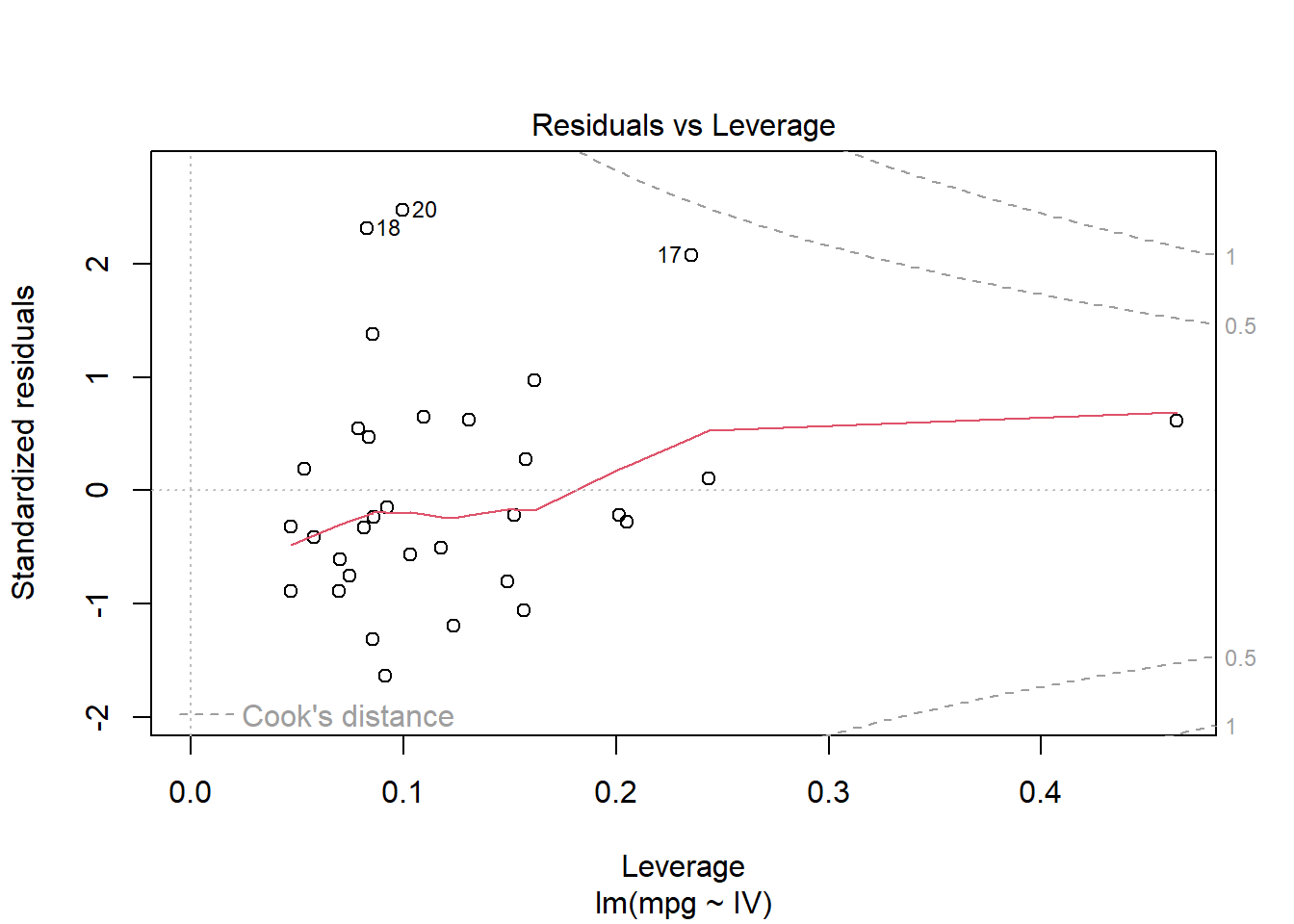lmtest::bptest(ols1)
##
##  studentized Breusch-Pagan test
##
## data:  ols1
## BP = 2.9351, df = 3, p-value = 0.4017
skedastic::white_lm(ols1) # White's Heteroscedasticity Test
## # A tibble: 1 × 5
##   statistic p.value parameter method       alternative
##       <dbl>   <dbl>     <dbl> <chr>        <chr>
## 1      5.83   0.442         6 White's Test greater
skedastic::breusch_pagan(ols1) # Breusch-Pagan Test
## # A tibble: 1 × 5
##   statistic p.value parameter method                alternative
##       <dbl>   <dbl>     <dbl> <chr>                 <chr>
## 1      2.94   0.402         3 Koenker (studentised) greater
skedastic::cook_weisberg(ols1) # Cook-Weisberg Test
## # A tibble: 1 × 5
##   statistic p.value parameter method alternative
##       <dbl>   <dbl>     <int> <chr>  <chr>
## 1      3.48   0.324         3 mult   greater
skedastic::glejser(ols1) # Glejser Test
## # A tibble: 1 × 4
##   statistic p.value parameter alternative
##       <dbl>   <dbl>     <dbl> <chr>
## 1      2.49   0.478         3 greater
skedastic::hetplot(ols1, horzvar = "fitted.values")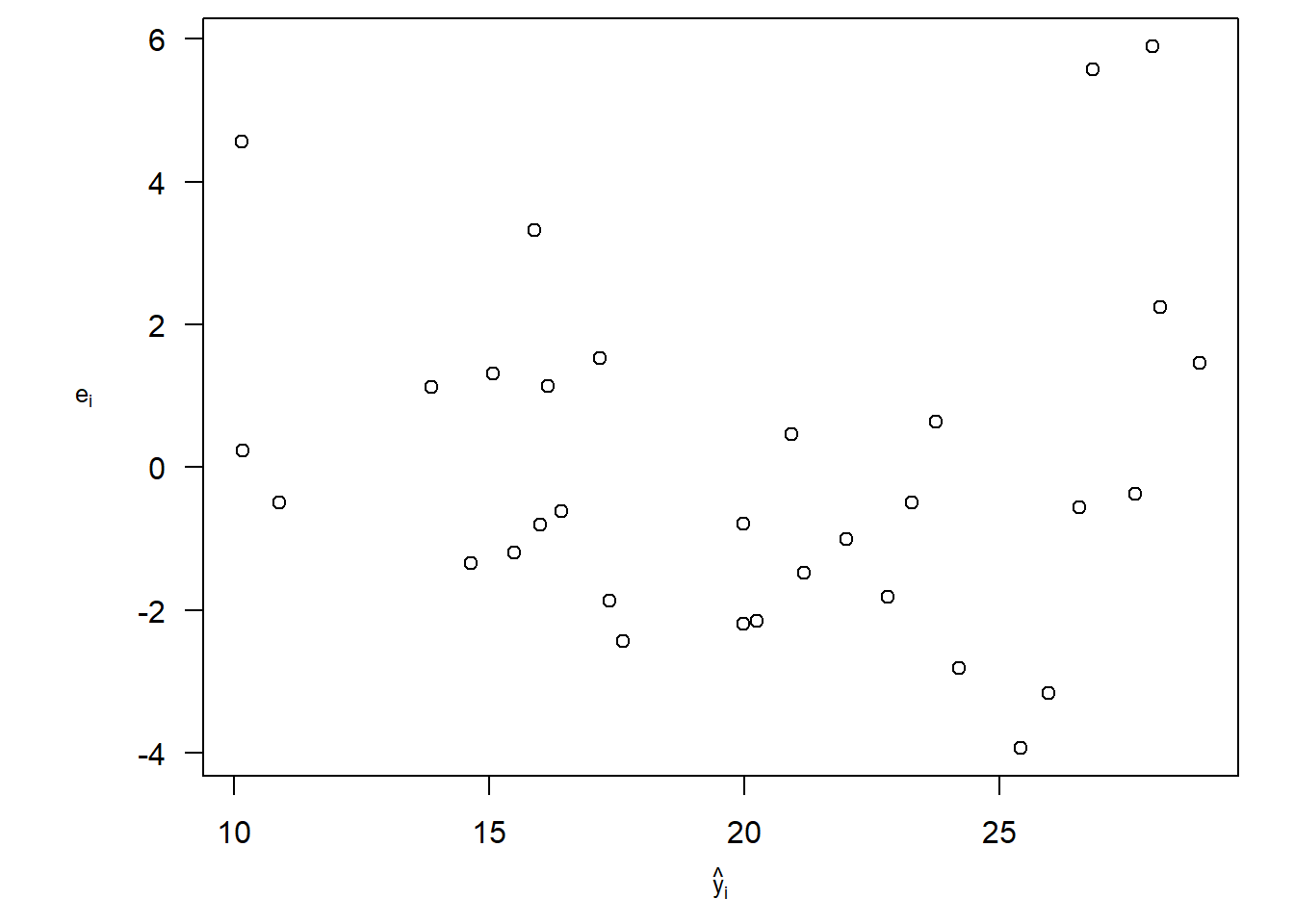Multicollinearity

To check for multicollinearity in the model, we can look at the correlations using the cor command.

Using the car package, we will estimate the Variance Inflation Factors (VIF). As a reule of thumb, multicollinearity is a serious problem if VIF > 10.

stargazer(cor(IV), type = "text", title = "Correlations")
##
## Correlations
## =====================
##      hp    cyl   wt
## ---------------------
## hp    1   0.832 0.659
## cyl 0.832   1   0.782
## wt  0.659 0.782   1
## ---------------------
vif1 <- vif(lm(mpg ~ hp + cyl + wt, data = ols))
stargazer(vif1, type = "text", title = "VIF")
##
## VIF
## =================
## hp     cyl   wt
## -----------------
## 3.258 4.757 2.580
## -----------------

Autocorrelation

Autocorrelation and Partial Autocorrelation plots are widely used in Econometrics. Here, acf and pacf commands are used on the residuals to determine their autocorrelation structure.

In addition to this, we can also apply the Durbin-Watson and Breusch-Godfrey test of serial correlation.

acf(error, lag.max = 6, type = "correlation")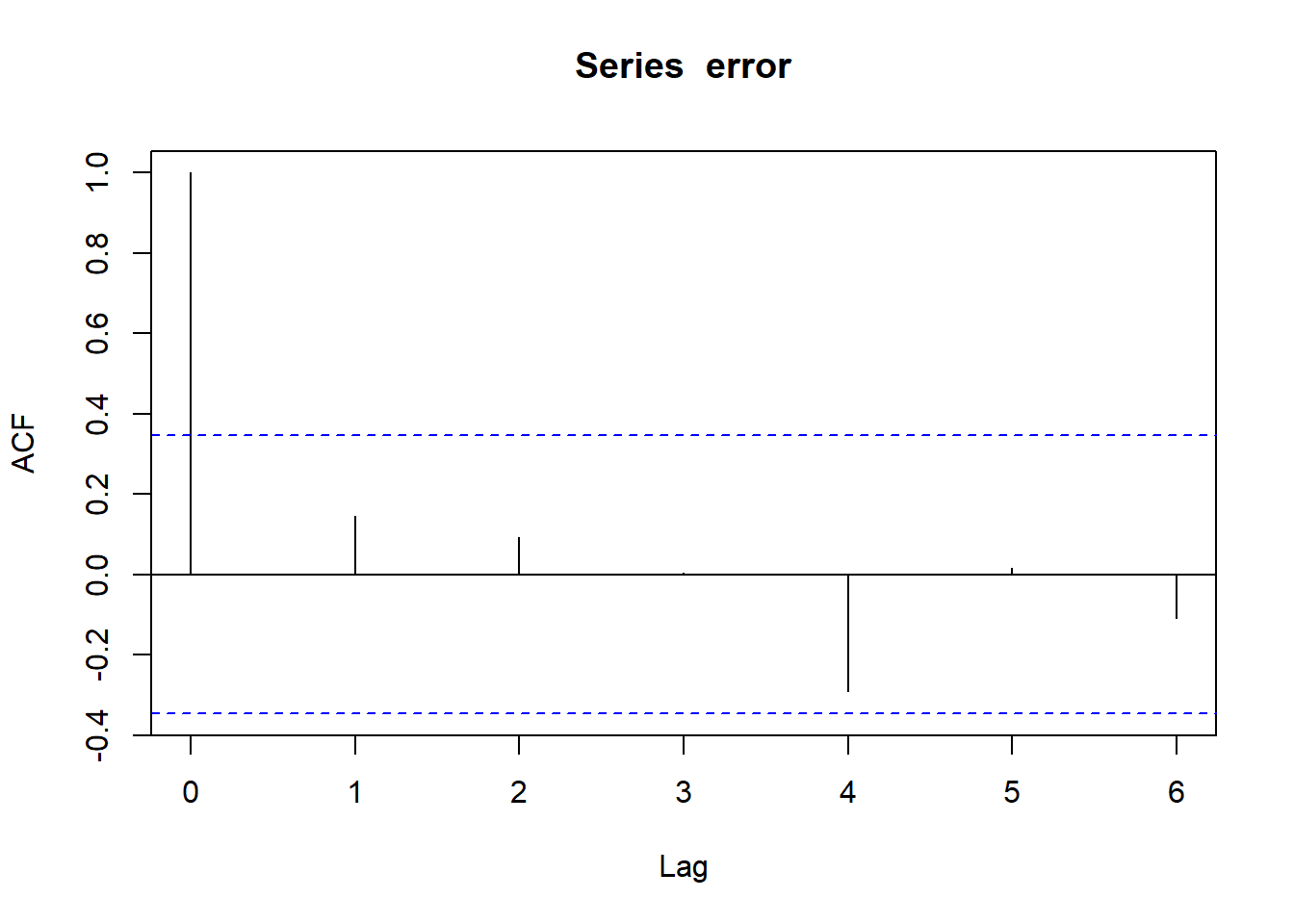pacf(error, lag.max = 6)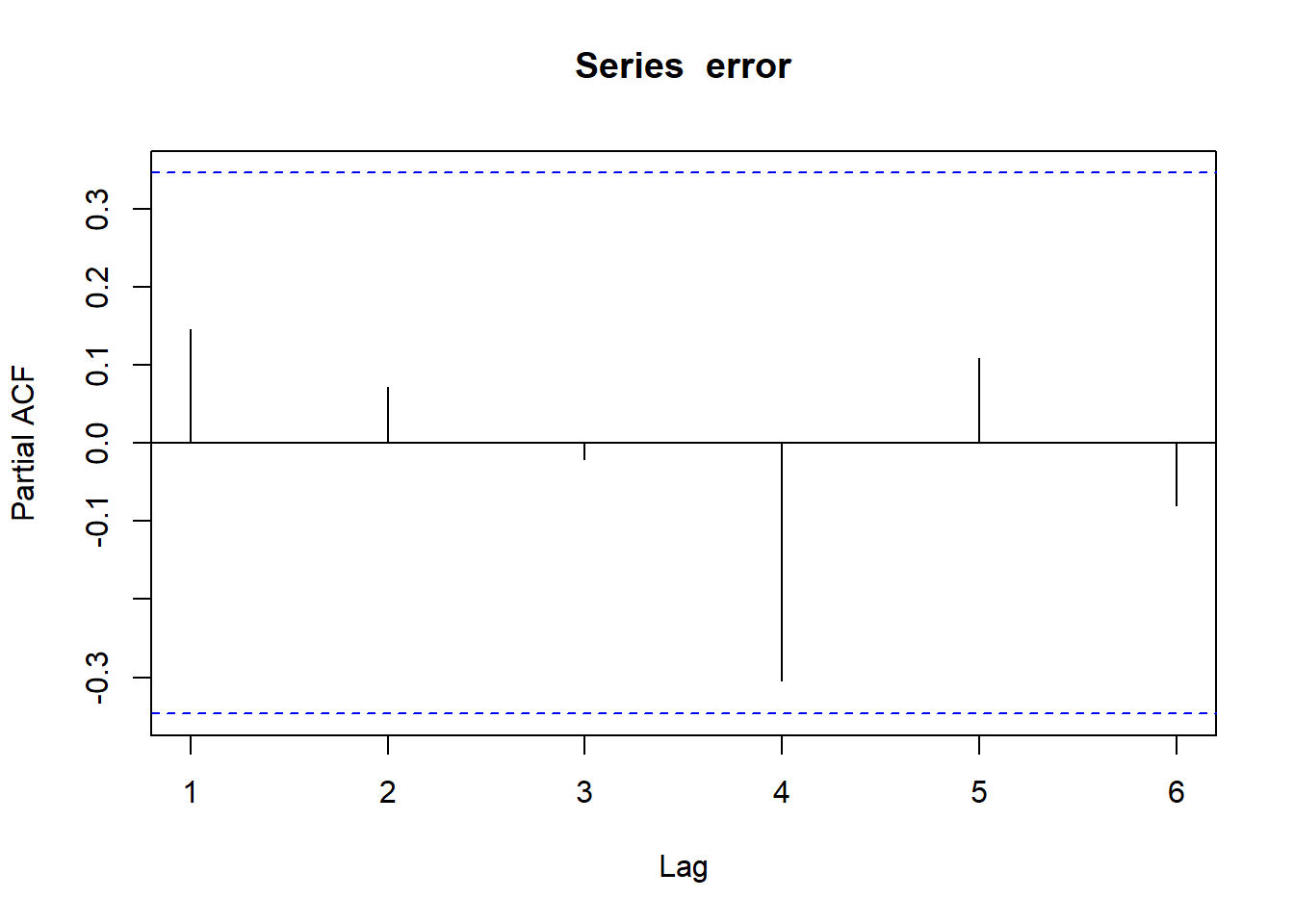lmtest::dwtest(ols1) # Durbin-Watson test for AR(1) only
##
##  Durbin-Watson test
##
## data:  ols1
## DW = 1.6441, p-value = 0.1002
## alternative hypothesis: true autocorrelation is greater than 0
lmtest::bgtest(ols1, order = 6)
##
##  Breusch-Godfrey test for serial correlation of order up to 6
##
## data:  ols1
## LM test = 5.0855, df = 6, p-value = 0.5329